# KSEEB Solutions for Class 10 Maths Chapter 13 Statistics Additional Questions

Students can Download Class 10 Maths Chapter 13 Statistics Additional Questions, Notes Pdf, KSEEB Solutions for Class 10 Maths helps you to revise the complete Karnataka State Board Syllabus and score more marks in your examinations.

## Karnataka State Syllabus Class 10 Maths Chapter 13 Statistics Additional Questions

I. Multiple Choice Questions:

Question 1.
The most frequently used measure of central tendency is
a. Mean
b. Mode
c. Median
d. None of these
a. MeanQuestion 2.
The item value which occurs most frequently is called
a. Mean
b. Median
c. Mode
d. None of these
c. Mode

Question 3.
The mean of first ten odd natural number is
a. 5
b. 10
c. 20
d. 19
b. 10

Question 4.
The mean of first n natural number is
a. $$\frac{n+1}{2}$$
b. $$\frac{n(n+1)}{2}$$
c. $$\frac{n(n-1)}{2}$$
d. n2
a. $$\frac{n+1}{2}$$

Question 5.
The class mark of the class interval 20 – 29 is
a. 20
b. 29
c. 25
d. 24.5
d. 24.5

Question 6.
In the distribution, the frequency of the class 0 – 5 is
a. 1
b.2
c. 3
d. 4
d. 4Question 7.
The median of the following series is 520, 20, 340, 190, 35, 800, 12, 10, 50, 80
a. 1210
b. 520
c. 190
d. 80
d. 80

Question 8.
If the mean of 5, 7, 9, x is 9 then the value of x is
a. 11
b. 15
c. 18
d. 16
b. 15

Question 9.
The mode of the distribution 3, 5, 7, 4, 2, 1, 4, 3, 4 is
a. 7
b. 4
c. 3
d. 1
b. 4

Question 10.
If the median of the following data arranged in ascending order is 18, then the value of x in 8, 11, 12, 16, 16 + x, 20, 25, 30 is
a. 1
b. 2
c. 3
d. 4
d. 4

Question 11.
The median of first 10 odd numbers is
a. 10
b. 8
c. 9
d. 11
a. 10

Question 12.
The mean of five numbers is 18. If one 19 number is removed, then the mean becomes 16. The removed number is
a. 22
b. 24
c. 25
d. 26
a. 22Question 13.
The mean of 13 numbers is 24. If 3 is added to each of the numbers. Then, the new mean is
a. 21
b. 24
c. 27
d. 30
c. 27

Question 14.
The mean of 1, 2, 3, 4, 5x is
a. x + 1
b. 5
c. 5x + 1
d. x + 2
d. x + 2

Question 15.
If the mean of 10 observations is 15, then their algebraic sum is
a. 150
b. 15
c. 1.5
d. 1500
a. 150

Question 16.
The mean of 10 numbers is 16. If one number 36 of these is changed to 26, then new mean is
a. 15
b. 16
c. 10
d. 26
a. 15

Question 17.
The mode of a frequency distribution can be determined graphically from.
a. histogram
b. frequency polygon
c. frequency curve
d. ogive
a. histogramQuestion 18.
If the mode of the following distribution is 2.8, then find the value of x. 2.5, 2.5, 2.1, 2.7, 2.8, 2.5, x, 2.8, 2.8, 2.7
a. 2.7
b. 2.5
c. 2.8
d. 2.1
c. 2.8

Question 19.
Which of the following is not a measure of central tendency.
a. Mean
b. Median
c. Mode
d. Range
d. Range

Question 20.
Relationship among mean, median and mode is
a. 3 Median = 2 Mode + Mean
b. 3 Median = 2 Mean + Mode
c. Mode = 2 Mean – Median
d. Mode = 3 Mean – 2 Median
b. 3 Median = 2 Mean + Mode

Question 21.
The mean and median of a data are respectively 20 and 22. The value of mode is
a. 20
b. 26
c. 22
d. 21
b. 26

Question 22.
The class, whose cumulative frequency is greater than (and nearest to) $$\frac{N}{2}$$, is called
a. average class
b. Modal class
c. Mean class
d. Median class
d. Median classQuestion 23.
The curve less than ogive in a cumulative frequency distribution is
a. decreasing
b. constant
c. Increasing
d. straight line
c. Increasing

Question 24.
The curve more than ogive in a cumulative frequency distribution is
a. decreasing
b. constant
c. increasing
d. straight line
a. decreasing

Question 25.
Which measure of central tendency is given by the x – coordinate of the point of intersection of the ‘more than ogive’ and ‘less than ogive’?
a. Mean
b. Median
c. Mode
d. Range
b. Median

Question 1.
Write the formula to find mean for grouped data direct method.
Mean =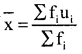Question 2.
Write the formula to find mean for grouped data using step deviation method.
Mean =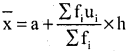Question 3.
Write the formula to find mode for grouped data
Mode =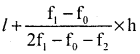Question 4.
Write the formula to find median for grouped data.
Median =Question 5.
Find the class mark of the class interval 30 – 40.
Class mark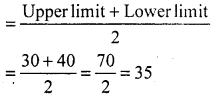Question 6.
Find the mean of the first 7 natural numbers.
Mean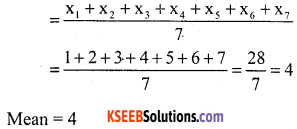Question 7.
Adata has 19 observations arranged in descending order which observation represents the median data?
Total number of observations = 19 (odd).
∴ Median will be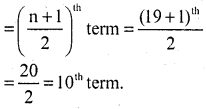10th term will be the median.

Question 8.
Write the modal class for the given frequency distribution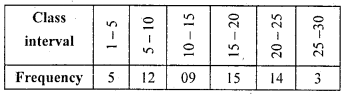Modal class is 15 – 20. It has highest frequency.Question 9.
Write the median class for the given frequency distribution.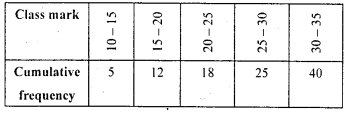Cumulative frequency > $$\frac{N}{2}=\frac{40}{2}$$ = 20 is 25.
Median class is 25 – 30

Question 10.
Write the empirical relationship between the three measures of central tendency?
3 median = mode + 2 mean

Question 1.
If the mean of the following distribution is 6, find the value of P.$$\overline{\mathbf{X}}$$ = 6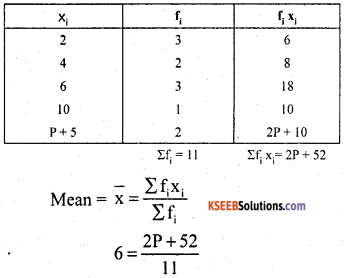66 = 2P + 52
2P = 66 – 52
2P = 14
P = $$\frac{14}{2}$$ = 7
P = 7

Question 2.
If xi‘s are the mid points of the class intervals of the class intervals of a grouped data, fi‘s are the corresponding
frequencies and $$\overline{\mathbf{X}}$$ is the mean, then find Σfi(xi – $$\overline{\mathbf{X}}$$).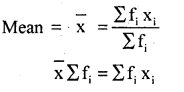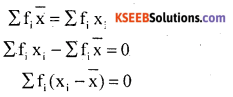Question 3.
The following table gives the literacy rate (in percentage) of 35 cities. Find the mean literacy rate.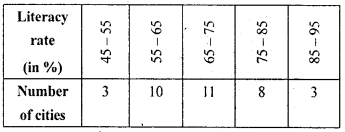Assumed mean = a = 70
Class size = h = 10∴ Mean literacy rate is 69.43%

Question 4.
A life insurance agent found the following data for distribution of ages of 100 policy holders. Calculate the median age, if policies are given only to persons having age 18 years onwards but less than 60 years.Given cumulative frequency distribution table convert into frequency distribution tableCumulative greater than $$\frac{\mathrm{n}}{2}=\frac{100}{2}$$ = 50 is 78.
∴ Median class is 35 – 40
l = 35, h = 5, f = 33 and cf=45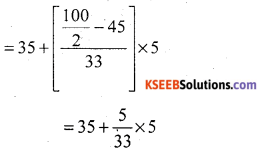= 35 + 0.76
= 35.76
∴ Median age is 35.76 years.

Question 5.
The mean of the following frequency table is 50. But the frequencies f1 and f2 in class 20 – 40 and 60 – 80 respectively are missing. Find the missing frequencies.Assumed mean = a = 50 and h = 20Σfi = 68 + f1 + f2 = 120
f1 + f2 = 120 – 68
f1 + f2 = 52 → (1)0 = 4 – f1 + f2.
f1 – f2 = 4 → (2)